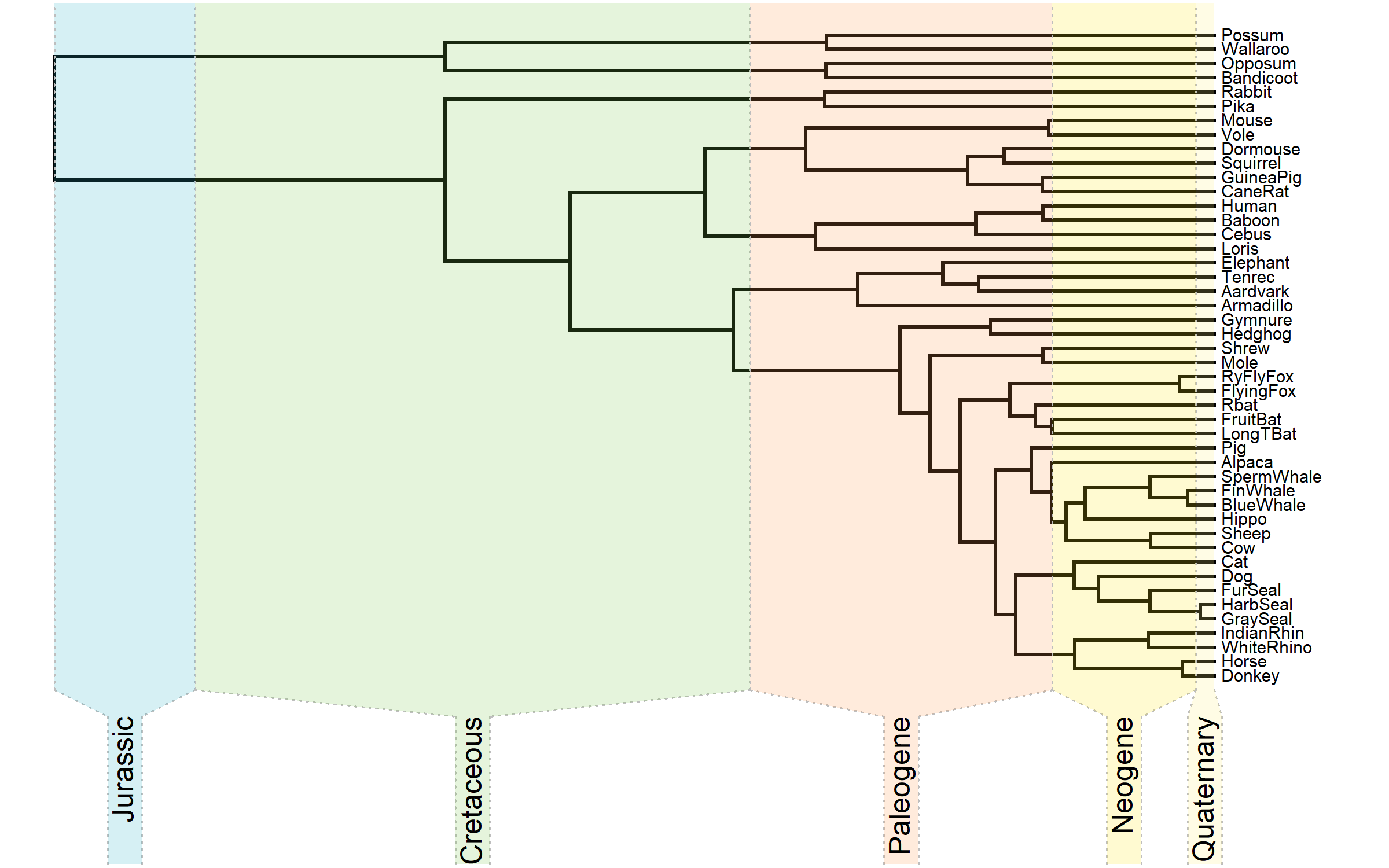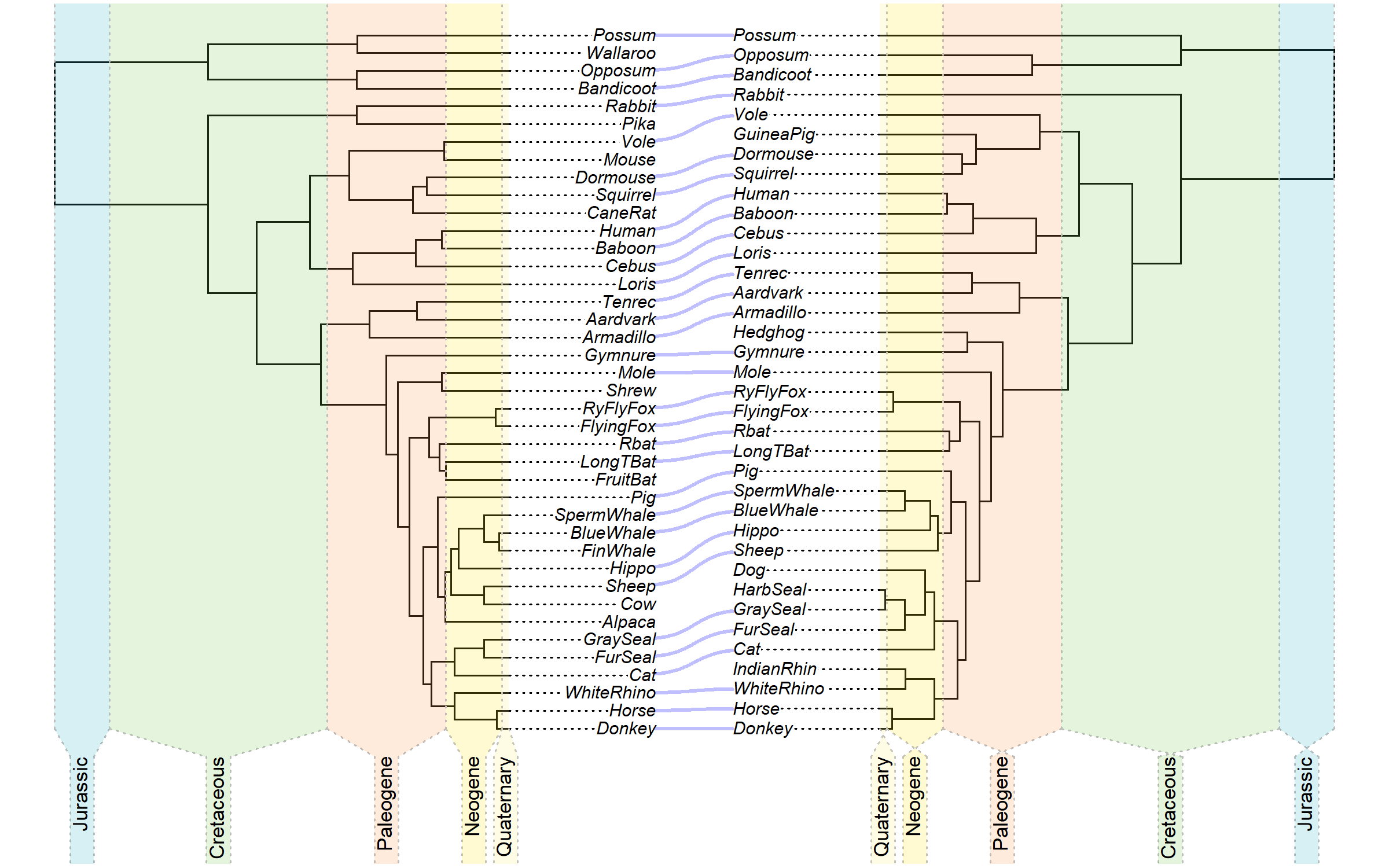## Friday, October 29, 2021

### Co-phylogenetic plot with geological colors

A couple of days ago, I received the following phytools user email:

“I wonder whether it would be possible to use `geo.legend` along with `cophylo`. Alternatively, if you know of any workaround to add a geological time scale to a `cophylo` plot object. Any help would be very much appreciated.”

Well, in R the question is never if but always how (to paraphrase Simone Blomberg) – so let's see how.

For this example, I'll use a single estimated tree obtained from the `Laurasiatherian` dataset in the phangorn package.

I'm going to set the divergence date separating marsupial & placental mammals at 165 mybp based on http://www.timetree.org/, and then (crudely) time-calibrate the tree using `chronos`.

``````library(phytools)
tree<-drop.tip(root(tree,"Platypus"),"Platypus")
tree<-chronos(tree,calibration=makeChronosCalib(tree,age.min=165,
age.max=165))
``````
``````##
## Setting initial dates...
## Fitting in progress... get a first set of estimates
##          (Penalised) log-lik = -17.00735
## Optimising rates... dates... -17.00735
##
## log-Lik = -17.00713
## PHIIC = 302.01
``````

Here's what this tree looks like with the default geological timescale overlain.

``````plotTree(tree,ylim=c(-10,Ntip(tree)),fsize=0.6)
geo.legend()
``````Now what I'm going to do is subsample the tips of this tree – just so that I have some incongruence between my left & right trees in the `cophylo` plot.

In a more realistic use-case, perhaps we'd have a time-calibrated tree for host & parasite species, and then table of associations between the two.

``````left.tree<-drop.tip(tree,sample(tree\$tip.label,6))
right.tree<-drop.tip(tree,sample(tree\$tip.label,10))
``````

Next, I'm going to create my plot. To do this, I'll first create & graph my `"cophylo"` object.

Then, I'll pull the `"last_plot.cophylo"` object that `plot.cophylo` creates and assign the `left` `"cophylo"` tree to a new object called `"last_plot.phylo"` that'll be used internally by `geo.legend`.

Then I'll make my geological colors palette using `geo.palette`. Actually, I could use a different set of time periods or colors if I wanted to – I'd just have to create a new table containing them.

Next, I'll rescale the time periods in my table to match those of my new plotting space.

This is critical because when we plot a `"cophylo"` object the space is no longer in the units of the edge lengths of our original tree!

Finally, I'll add the geological period legend using `geo.legend` – and then simply repeat the same thing for my other other plotted tree.

``````## plot cophylo
plot(cophylo(left.tree,right.tree),ylim=c(-0.15,1),fsize=0.6,
pts=FALSE,part=0.47)
``````
``````## Rotating nodes to optimize matching...
## Done.
``````
``````## pull out the last plotted cophylo
obj<-get("last_plot.cophylo",envir=.PlotPhyloEnv)
## reset some attributes of the last plotted cophylo
tmp<-obj\$left
tmp\$direction<-FALSE
tmp\$Ntip<-1
## assign it to last_plot.phylo
assign("last_plot.phylo",tmp,envir=.PlotPhyloEnv)
## extract geological color palette using geo.palette
pal<-geo.palette()
## find the time period that matches the depth of the
## original tree
ii<-which(pal\$period[,1]>max(nodeHeights(left.tree)))
## subsample the geological palette matrix
pal\$period[ii,1]<-max(nodeHeights(left.tree))
pal\$colors<-pal\$colors[1:ii]
## find the x-limits of the tree
XLIM<-range(tmp\$xx)
## rescale to these new limits
new.pal<-XLIM-pal\$period/max(nodeHeights(left.tree))*diff(XLIM)
geo.legend(leg=new.pal,colors=pal\$colors,cex=0.7)
## repeat for the other tree
tmp<-obj\$right
tmp\$direction<-FALSE
tmp\$Ntip<-1
assign("last_plot.phylo",tmp,envir=.PlotPhyloEnv)
pal<-geo.palette()
ii<-which(pal\$period[,1]>max(nodeHeights(right.tree)))
pal\$period[ii,1]<-max(nodeHeights(right.tree))
pal\$colors<-pal\$colors[1:ii]
XLIM<-range(tmp\$xx)
new.pal<-pal\$period/max(nodeHeights(tree))*diff(XLIM)+XLIM
geo.legend(leg=new.pal,colors=pal\$colors,cex=0.7)
``````That's it.